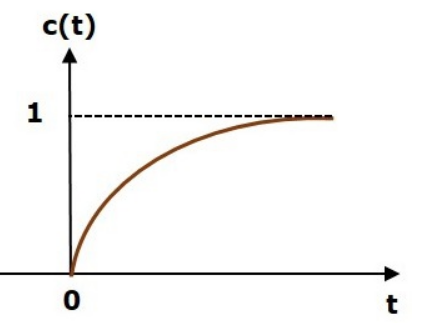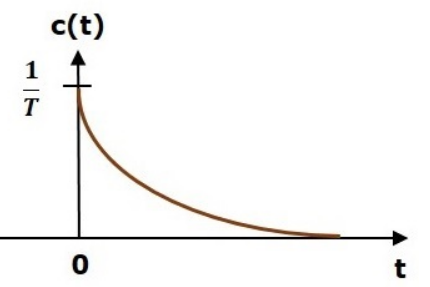### Unit Step Response of 1st order system

Unit Step Response of 1st order system:
Unit step signal is used as input signal i.e r(t)=u(t)=1; for t>=0. Where r(t) is the input signal and u(t) is the unit step signal.
Applying laplace transform we get R(s) .
Substituting R(s) in the equation we get

Applying inverse laplace transform to the above equation we get,

Where T is the Time Constant and denotes the time required by the system to attain the 63.2% of the steady state value.Time Constant(T) indicates how fast the system reaces the steady state.Smaller the Time Constant(T), faster the system.
Plotting the time response we get the following:Impulse Response of 1st order system:
For impulse time response we use the impulse signal as the input. I.e r(t)=(t)
Applying laplace transform we get,
R(s)= 1
Substituting R(s) in transfer function equation we get,

Taking inverse laplace transform we get,

Plotting time response we get,Ramp Response for a 1st order system:
We use unit ramp signal as input to get the rap response of the system.
I.e r(t)= tu(t)
Applying Laplace transform,

Substituting R(s) in Transfer Function equation,

Applying inverse laplace transform we get,

Time Response:As we can see that the output we get differs from the actual output from a difference of T which is known as the steady state error.
Comparison:
When we compare the above three time response we can see that the unit step response is the derivative of ramp response and the impulse response is the derivative of the unit step response.
Above behaviour is the property of a Linear Time-Invariant(LTI) System.